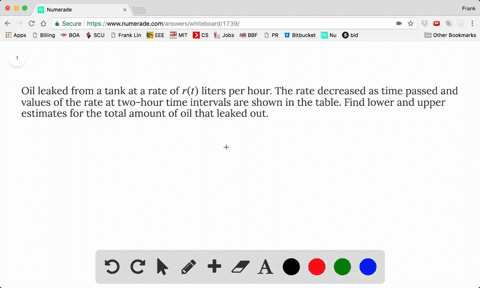### Oil leaked from a tank at a rate of $r(t)$ lite…

01:24
FL
Problem 14

The table shows a speedometer readings at 10-second intervals during a 1-minute period for a car racing at the Daytona International Speedway in Florida.

(a) Estimate the distance the race car traveled during this time period using the velocities at the beginning of the time intervals.

(b) Give another estimate using the velocities at the end of the time periods.

(c) Are your estimates in parts (a) and (b) upper and lower estimates? Explain.

(A). $\approx 2.38$ miles
(B). $\approx 2.36$ miles
(C). Upper Estimate: $L_{6}$ Lower Estimate: $R_{6}$

## Discussion

You must be signed in to discuss.

## Video Transcript

And this problem you have You have a table that helps you. Ah, uh, the one minute period ofthe car racing and so s made a distance by using the using the velocity at beginning offish time and in the violence has end of each time interval and ask you the last question. So for if you part you speaking we all the time and so each each time intervals ten seconds. So we put a tent here and we just have to some other velocities here. Ah, if one eighty two point nine plus, you have the value for table right plus one, sixty eight point zero plus one hundred six point six plus ninety nine point eight plus one twenty four point five plus one seventy six point one. Ah, But here as you and be careful that Ah, you'LL have to Ah, divide by thirty six hundred if you want to put the your union of smiles people So this time's measuring second and the thiss This philosophy is measured in miles per hour. So it's ours thirty six hundred seconds. So that's why I say And you have to divide by this a similar for Poppy This is your velocity, sir. This is your time off each time interval and we use the end. Like you said, the velocity as the end of time periods this estimate. So you just add up off them. Look at these value you can find on tech support. And for a similar reason you have divided by seriously House six hundred took as a unit, miles. And is this opera and overestimates well on its neither off them Because offering the lower estimate means means you use the largest and the smallest velocity at each time interval, for your estimation. And if we use beginning comment and pine and time eyes, neither off them.